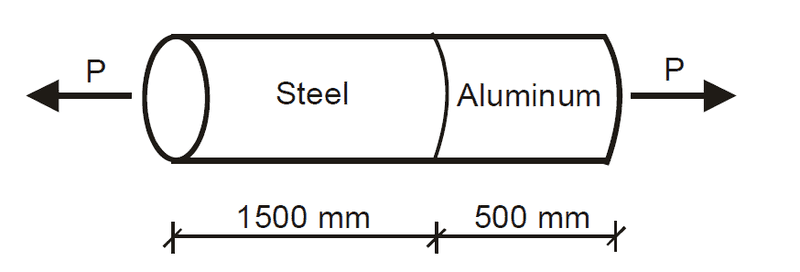# Finding normal stress in composite bar

newhere

## Homework Statement

The 2000 mm long composite bar shown in Fig. 1 consists of an aluminum bar having
a modulus of elasticity EAl = 70 GPa and length LAl = 500 mm, which is securely fastened
to a steel bar having modulus of elasticity ESt = 210 GPa and length LSt = 1500 mm. After
the force P is applied, a tensile normal strain of εAl = 1000 × 10-6 is measured in the
aluminum bar. Find the tensile normal stress in each bar and the total elongation of the
composite bar.ε_x=σ_x/E

## The Attempt at a Solution

So I first took the equation and rearranged it, such that ε_x*E=σ_x and got σ_AL=0.07 GPa. Then for the changed in distance of the aluminum bar, dl=ε*l_0 = (1000*10^-6)(500mm) = 0.5mm change for Al bar.

Assuming that's correct, I'm stuck on how to find the stress for the steel bar and the length it changed.

Homework Helper
Gold Member
The cross section area of each section is the same. If you know the stress in the alum piece, what must be the stress in the steel piece? Hint: what is the force in each piece as a function of P?

newhere
The cross section area of each section is the same. If you know the stress in the alum piece, what must be the stress in the steel piece? Hint: what is the force in each piece as a function of P?

So the stress would be the same in the steel piece?